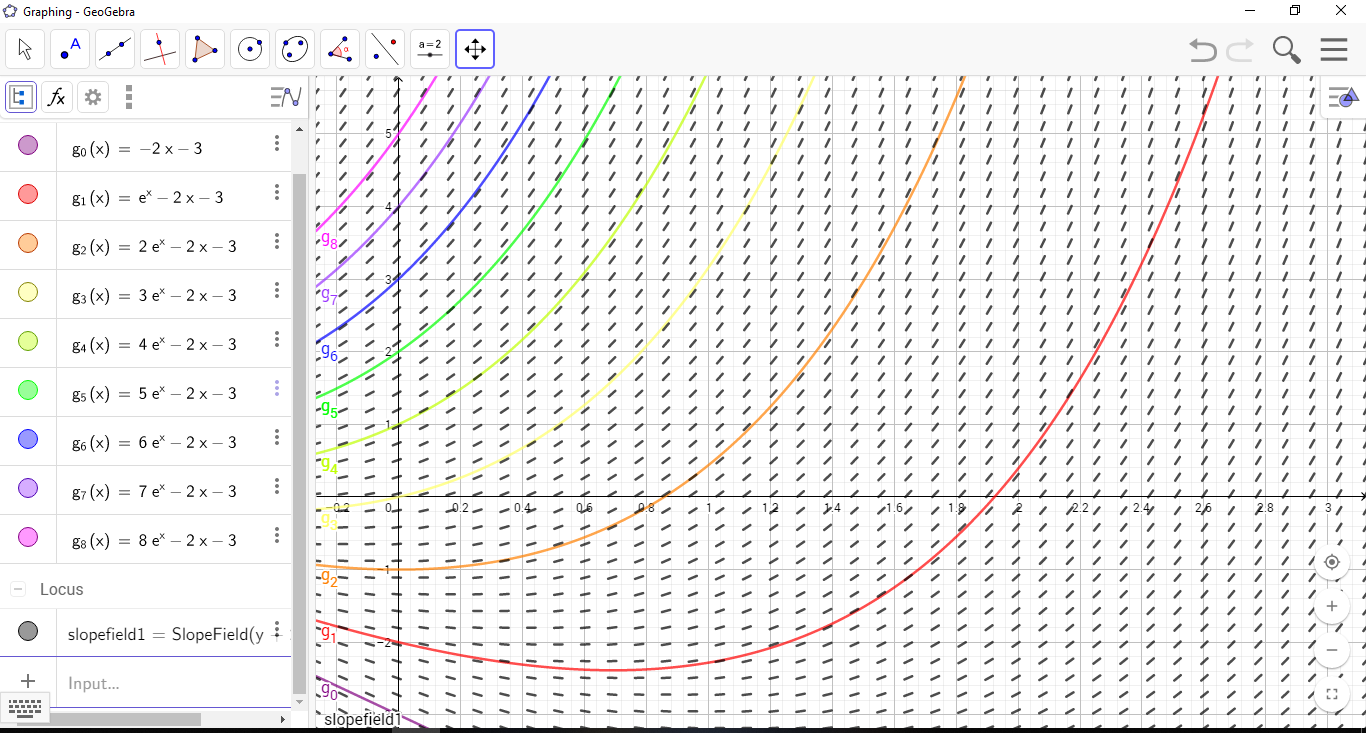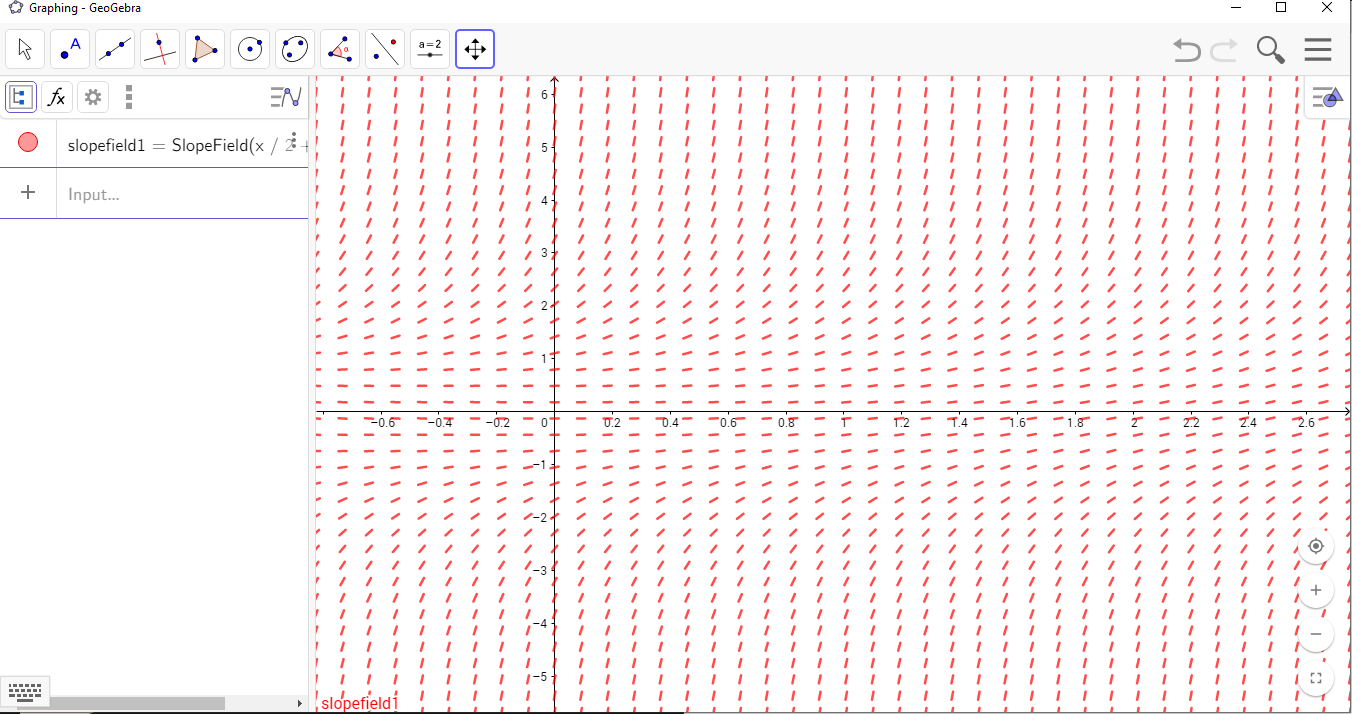# What Are Integral Curves and Direction Fields Used for?

## Integral CurvesAn integral curve—also known as a parametric curve—is the graph of a particular solution of a differential equation—that is, a solution where the constants are determined.

You can create these curves for particular solutions of both first and second order differential equations. If you have a collection of integral curves with different particular solutions, they are called a family of curves.

## Direction FieldsIf you have a differential equation of the first order, you can find the tangent at any point $\left(x,y\right)$. You do this by moving terms around so that ${y}^{\prime }$ ends up alone on the left-hand side of the equation. This gives you a formula where you can enter $x$ and $y$ into the formula for ${y}^{\prime }$. If you do this for all the points in a region you get a direction field or slope field.

This direction field also shows which direction the integral curve would go for those specific points. You can say how $y$ develops from a point $\left(x,y\right)$ by looking at which direction the direction field shows at that point $\left(x,y\right)$.